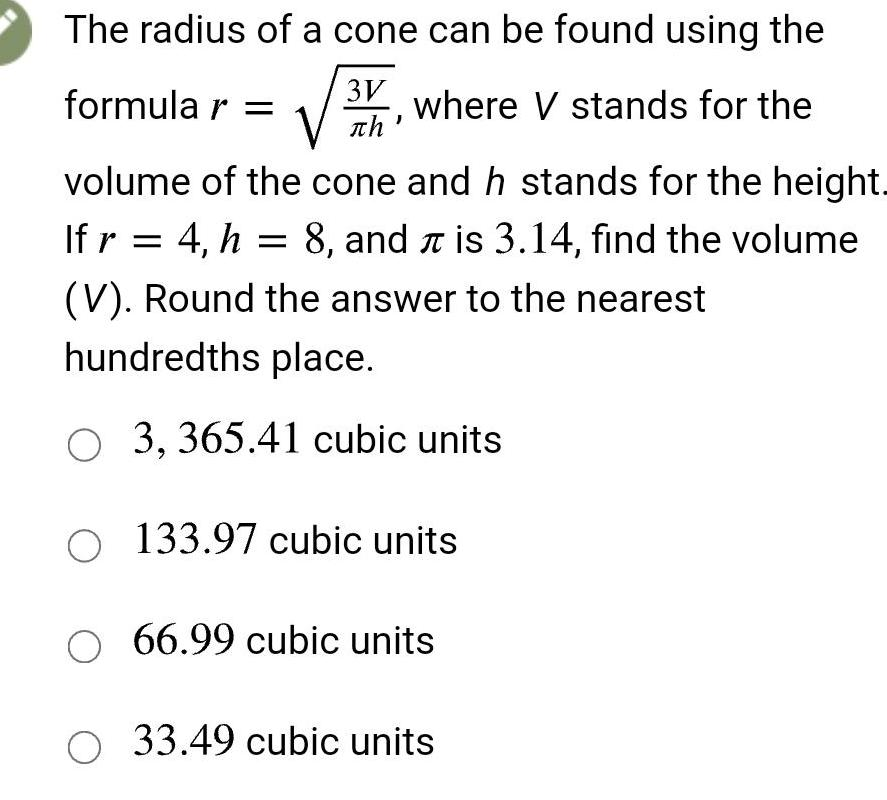Question:

# The radius of a cone can be found using the formular 3V

Last updated: 3/10/2023The radius of a cone can be found using the formular 3V where V stands for the th volume of the cone and h stands for the height Ifr 4 h 8 and is 3 14 find the volume V Round the answer to the nearest hundredths place O3 365 41 cubic units O 133 97 cubic units O 66 99 cubic units O 33 49 cubic units